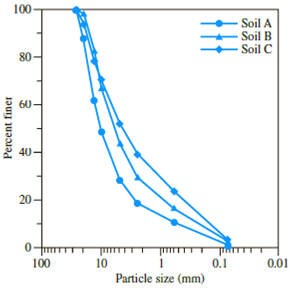Chapter 2, Problem 2.1CTPPrinciples of Geotechnical Enginee...

9th Edition
Braja M. Das + 1 other
ISBN: 9781305970939

Solutions

Chapter
SectionPrinciples of Geotechnical Enginee...

9th Edition
Braja M. Das + 1 other
ISBN: 9781305970939
Textbook Problem

Three groups of students from the Geotechnical Engineering class collected soil-aggregate samples for laboratory testing from a recycled aggregate processing plant in Palm Beach County, Florida. Three samples, denoted by Soil A, Soil B, and Soil C, were collected from three locations of the aggregate stockpile, and sieve analyses were conducted (see Figure 2.35).(a)(b)Figure 2.35 (a) Soil-aggregate stockpile; (b) sieve analysis (Courtesy of Khaled Sobhan, Florida Atlantic University, Boca Raton, Florida)a. Determine the coefficient of uniformity and the coefficient of gradation for Soils A, B, and C.b. Which one is coarser: Soil A or Soil C? Justify your answer.c. Although the soils are obtained from the same stockpile, why are the curves so different? (Hint: Comment on particle segregation and representative field sampling.)d. Determine the percentages of gravel, sand and fines according to Unified Soil Classification System.

(a)

To determine

Calculate the coefficient of uniformity (Cu) and coefficient of gradation (Cc) for soils A, B, and C.

Explanation

Sketch the grain size distribution curve for soils A, B, and C as shown in Figure 1.

Refer to Figure 1.

For soil A:

The diameter of the particle corresponding to 60% finer (D60) is 14mm.

The diameter of the particle corresponding to 30% finer (D30) is 5.2mm.

The diameter of the particle corresponding to 10% finer (D10) is 0.53mm.

For soil B:

The diameter of the particle corresponding to 60% finer (D60) is 8.1mm.

The diameter of the particle corresponding to 30% finer (D30) is 2.5mm.

The diameter of the particle corresponding to 10% finer (D10) is 0.23mm.

For soil C:

The diameter of the particle corresponding to 60% finer (D60) is 7mm.

The diameter of the particle corresponding to 30% finer (D30) is 1.1mm.

The diameter of the particle corresponding to 10% finer (D10) is 0.15mm.

Calculate the uniformity coefficient (Cu) using the relation.

Cu=D60D10 (1)

For soil A:

Substitute 14mm for D60 and 0.53mm for D10 in Equation (1).

Cu=140.53=26.42

Hence, the uniformity coefficient for soil A is 26.42_.

For soil B:

Substitute 8.1mm for D60 and 0.23mm for D10 in Equation (1).

Cu=8.10.23=35

Hence, the uniformity coefficient for soil B is 35_

(b)

To determine

State which of the soil is coarser from soil A and C.

(c)

To determine

Explain the reason for curve different of soil A, B and C if it is obtained from same stockpile.

(d)

To determine

Calculate the percentages of gravel, sand, and fines according to the Unified Soil Classification System.

Still sussing out bartleby?

Check out a sample textbook solution.

See a sample solution

The Solution to Your Study Problems

Bartleby provides explanations to thousands of textbook problems written by our experts, many with advanced degrees!

Get Started

What is the difference between vulnerability and exposure?

Principles of Information Security (MindTap Course List)

What is another name for the Cartesian coordinate system?

Precision Machining Technology (MindTap Course List)

5. Identify the main components of an information system. What is a mission-critical system?

Systems Analysis and Design (Shelly Cashman Series) (MindTap Course List)

Most users today work with a command-line interface.

Enhanced Discovering Computers 2017 (Shelly Cashman Series) (MindTap Course List)

What does the AWS abbreviation “SENSE” stand for?

Welding: Principles and Applications (MindTap Course List)